# 词频统计

def found_file(file_path):   file_name = open(file_path, "r", encoding="utf-8")   file_txt = file_name.read()   spce = re.compile(u'!@$#%&*,.，.?;:') file_txt = re.sub(spce,'',file_txt) file_txt = file_txt.lower() return file_txt.split()   功能1 小文件输入。 为表明程序能跑，结果真实而不是迫害老五，请他亲自键 盘在控制台下输入命令。 运行结果如下：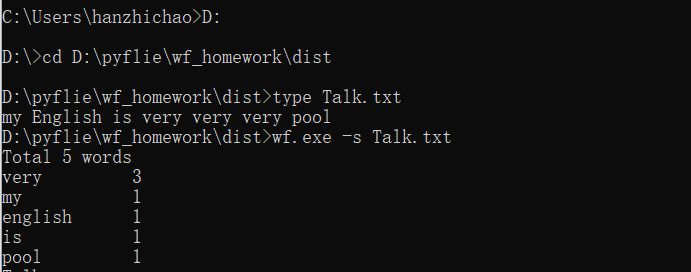这两个函数分别用于将单词存放在字典里，输出单词的个数，并且统计前十个单词的频次，第二个函数查找文件是否在路径之内并返回它的路径。 def put_num(word_list,f = True): d = {} for list in word_list: d[list] = d.get(list,0)+1 d_list = sorted(d.items(), key=lambda x: x, reverse=True) print("Total",len(d_list),"words") for i in range(min(len(d_list),10)): print("{0:<9}{1:>5}".format(d_list[i], d_list[i])) if (f == False): print("----") return Nonedef d_file(file_path): if (os.path.isdir(file_path) == True): return os.listdir(file_path) elif os.path.isfile(file_path) == True: return [file_path] return None 重定向代码： def function_four(file_txt): spce = re.compile(u'!@$#%&*,.，.?;:')   file_txt = re.sub(spce, '', file_txt)   file_txt = file_txt.lower()   file_txt =file_txt.split()   put_num(file_txt)   return  None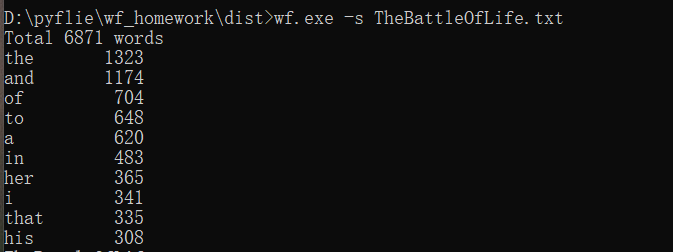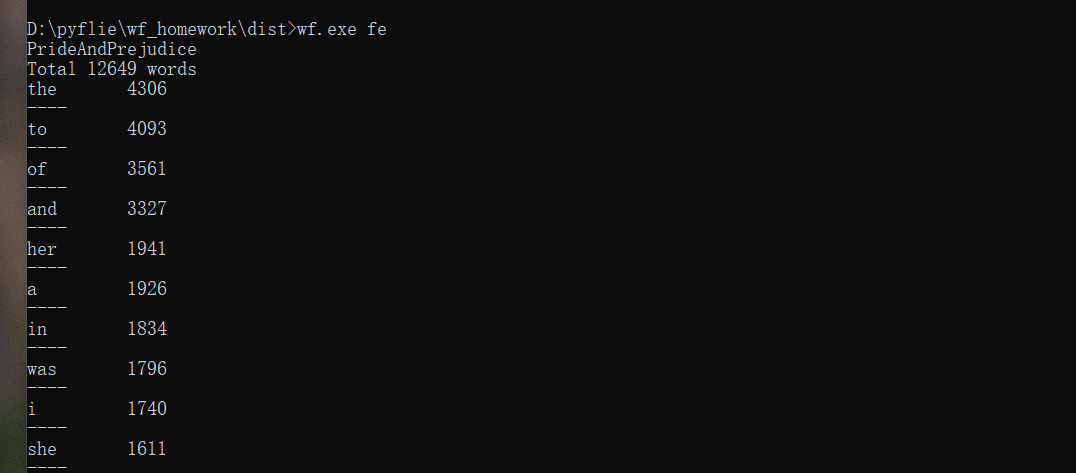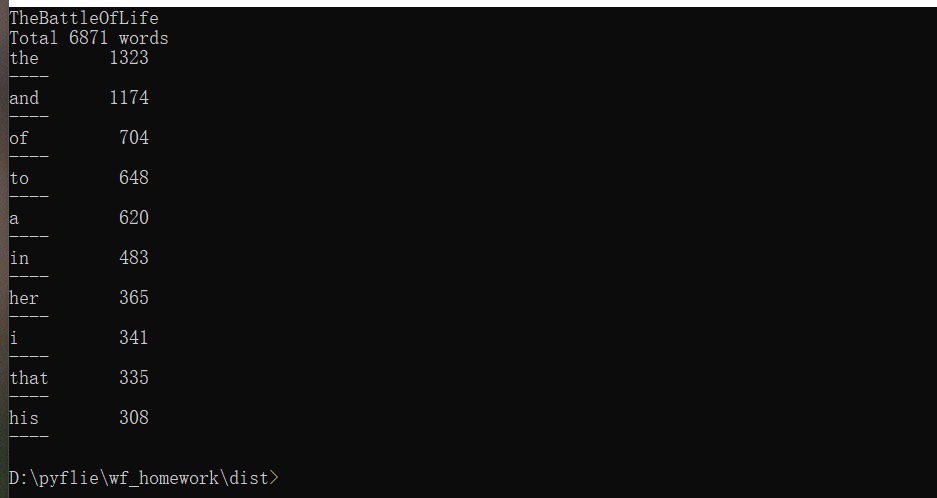功能4 从控制台读入英文单篇作品，这不是为了打脸老五，而是为了向你女朋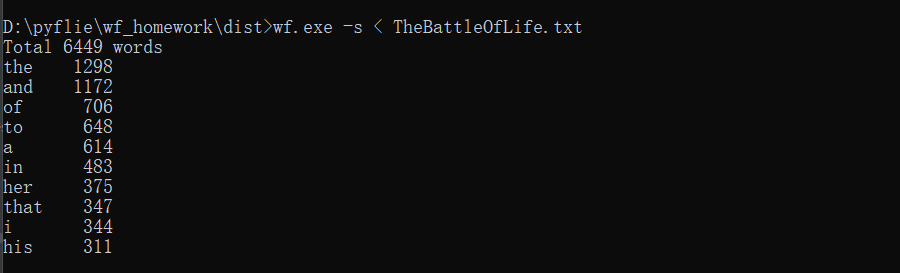PSP：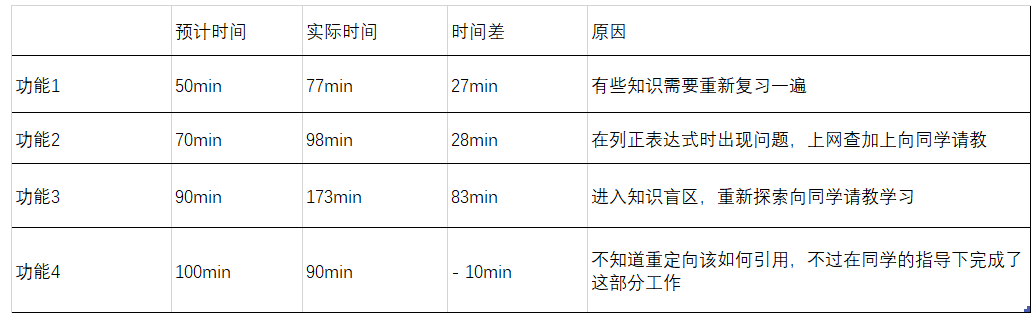posted @ 2020-09-23 08:34  我刀呢？  阅读(58)  评论(0编辑  收藏